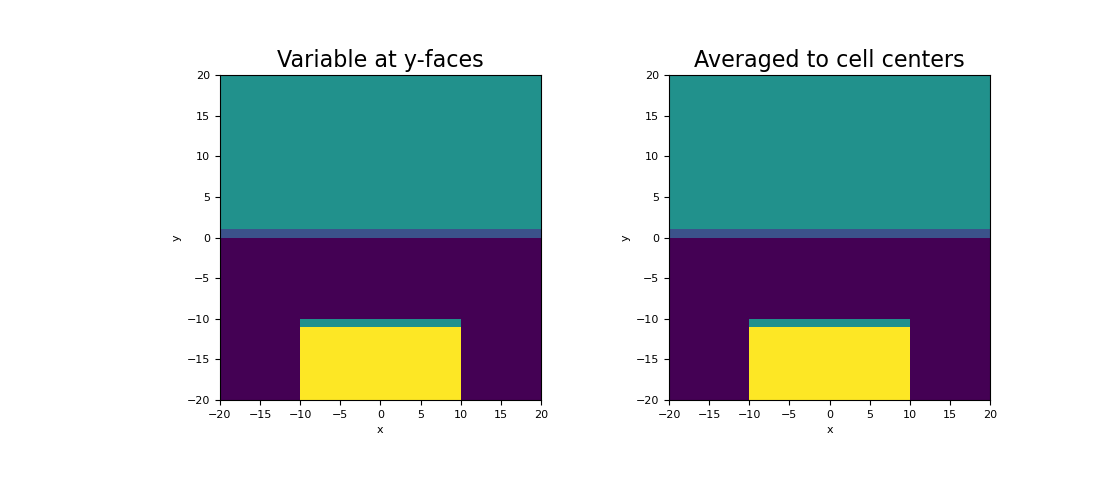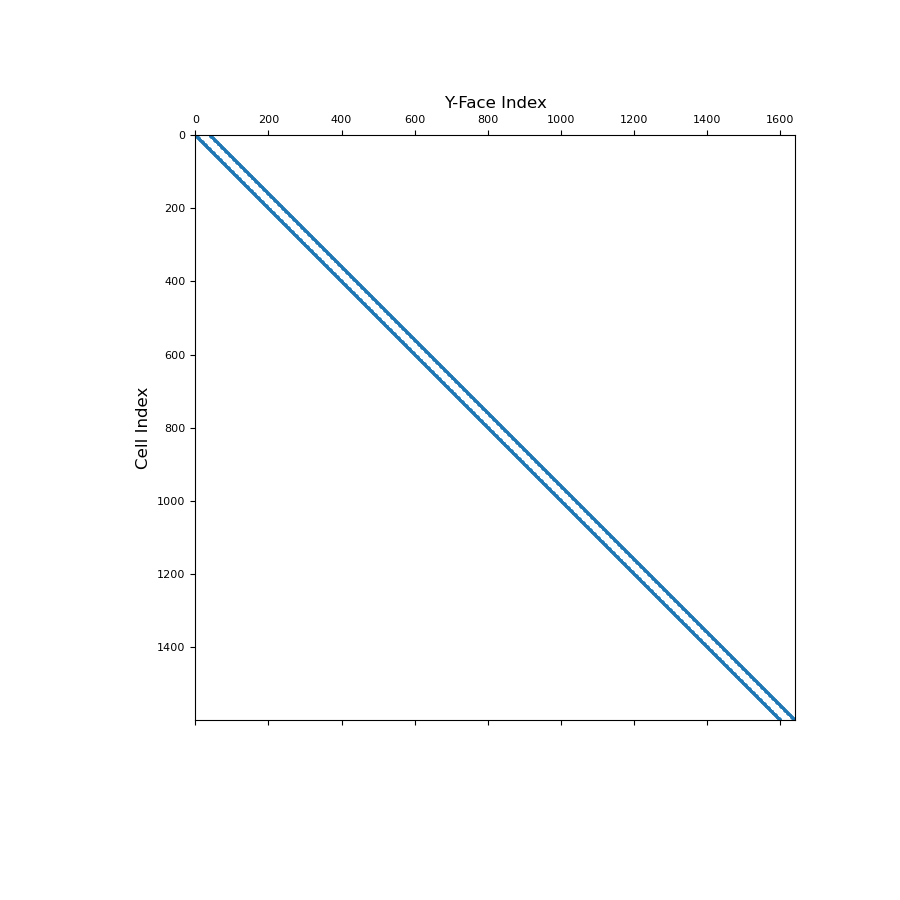# discretize.operators.DiffOperators.average_face_y_to_cell¶

property DiffOperators.average_face_y_to_cell

Averaging operator from y-faces to cell centers (scalar quantities).

This property constructs a 2nd order averaging operator that maps scalar quantities from y-faces to cell centers. This averaging operator is used when a discrete scalar quantity defined on x-faces must be projected to cell centers. Once constructed, the operator is stored permanently as a property of the mesh. See notes.

Returns
(n_cells, n_faces_y) scipy.sparse.csr_matrix

The scalar averaging operator from y-faces to cell centers

Notes

Let $$\boldsymbol{\phi_y}$$ be a discrete scalar quantity that lives on y-faces. average_face_y_to_cell constructs a discrete linear operator $$\mathbf{A_{yc}}$$ that projects $$\boldsymbol{\phi_y}$$ to cell centers, i.e.:

$\boldsymbol{\phi_c} = \mathbf{A_{yc}} \, \boldsymbol{\phi_y}$

where $$\boldsymbol{\phi_c}$$ approximates the value of the scalar quantity at cell centers. For each cell, we are simply averaging the values defined on its y-faces. The operation is implemented as a matrix vector product, i.e.:

phi_c = Ayc @ phi_y


Examples

Here we compute the values of a scalar function on the y-faces. We then create an averaging operator to approximate the function at cell centers. We choose to define a scalar function that is strongly discontinuous in some places to demonstrate how the averaging operator will smooth out discontinuities.

We start by importing the necessary packages and defining a mesh.

>>> from discretize import TensorMesh
>>> import numpy as np
>>> import matplotlib.pyplot as plt

>>> h = np.ones(40)
>>> mesh = TensorMesh([h, h], x0="CC")


Create a scalar variable on y-faces,

>>> phi_y = np.zeros(mesh.nFy)
>>> xy = mesh.faces_y
>>> phi_y[(xy[:, 1] > 0)] = 25.0
>>> phi_y[(xy[:, 1] < -10.0) & (xy[:, 0] > -10.0) & (xy[:, 0] < 10.0)] = 50.0


Next, we construct the averaging operator and apply it to the discrete scalar quantity to approximate the value at cell centers.

>>> Ayc = mesh.average_face_y_to_cell
>>> phi_c = Ayc @ phi_y


And finally, plot the results:

Expand to show scripting for plot
>>> fig = plt.figure(figsize=(11, 5))
>>> v = np.r_[np.zeros(mesh.nFx), phi_y]  # create vector for plotting function
>>> mesh.plot_image(v, ax=ax1, v_type="Fy")
>>> ax1.set_title("Variable at y-faces", fontsize=16)
>>> mesh.plot_image(phi_c, ax=ax2, v_type="CC")
>>> ax2.set_title("Averaged to cell centers", fontsize=16)
>>> plt.show()Below, we show a spy plot illustrating the sparsity and mapping of the operator

Expand to show scripting for plot
>>> fig = plt.figure(figsize=(9, 9))